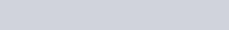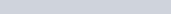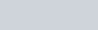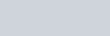• 1
•
•
•
•
•
•
•
1
Share

# TERMINAL VELOCITY

So in today’s article, we are going to talk about, the concept of terminal velocity. It is a very important and interesting phenomenon of hydrodynamics.
Everyone must have seen that when a stone and any other objects, fell in the pond or a well and any other water bodies, then the speed of falling of stone in water has been changed and start falling with a constant velocity.So, the question is, why the velocity of stone has changed and acquired a different velocity?
Then the answer to this question is explained below:

According to the Archimedes principle, if any object is immersed fully or partially in the fluid then that object experiences a buoyant force and this buoyant force is equal to the weight of fluid which is displaced by the object at the time of immersing. Every object displaced the same amount of volume as the volume of the object.

When a stone is dropped into the water, the buoyant force starts acting in the opposite direction of the falling object. But only Archimedes’s principle is not responsible for attaining this constant velocity.

We know that only ideal fluid is non-viscous, otherwise, all fluids are vicious to some extent. And we know that viscosity is the property of the fluid, which comes due to the friction between the two laminae which opposes the relative velocity of each other. And due to this friction between the two laminae of fluid, a force is generated, and this force is called the force of viscosity, also called dragging force. Which is derived by George G. Stokes. Which is famous as STOKES LAW.

It means when a piece of stone is dropped into the fluid of viscosity η then each lamina or layer also opposes the velocity of the falling object. And the force of viscosity is directly proportional to the viscosity of the fluid, if the fluid has higher viscosity then the force of viscosity will be also higher.

And higher viscous force than higher opposition. So if any object is dropped into the fluid of viscosity η then its motion is hindered or opposed by the two forces are buoyant force and viscous force. Both forces changed the velocity of the falling object to the constant Velocity, and that constant velocity is called terminal velocity.

If we formally define terminal velocity, we can say that the maximum constant velocity acquired by the falling object is the viscous fluid is terminal velocity. Now we are going to derive the formula for terminal velocity.

## DERIVATION FOR THE TERMINAL VELOCITY

Let’s take a spherical object of radius R and density ρ and density of the fluid is σ and its viscosity is η. If an object is in the fluid then the volume is displaced is equal to the volume of the spherical object.Putting all the values, we get-And the force of viscosity is given as-Then the weight of the object due to gravity is-In an ideal condition, The Buoyant force and viscous force balance the weight of the object, then-Canceling R and π on both sides we get the required terminal velocity as follow-This velocity is acquired by the object when they start falling in the fluid.

Note: This formula depends on the shape, size, and geometry of the object. If geometry changes then the formula is also changed. Because this formula depends on the fluid which displaced, but we know that cubicle object displaced different volume of fluid, conical, cylindrical, cuboidal also displaced different volume of fluid. For this, there are different terminal velocities. This terminal velocity is only for spherical objects.